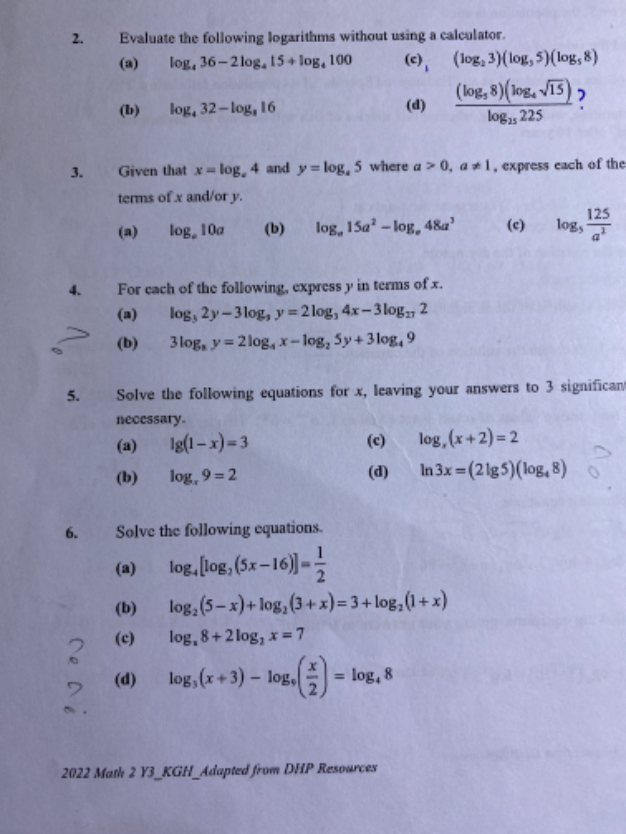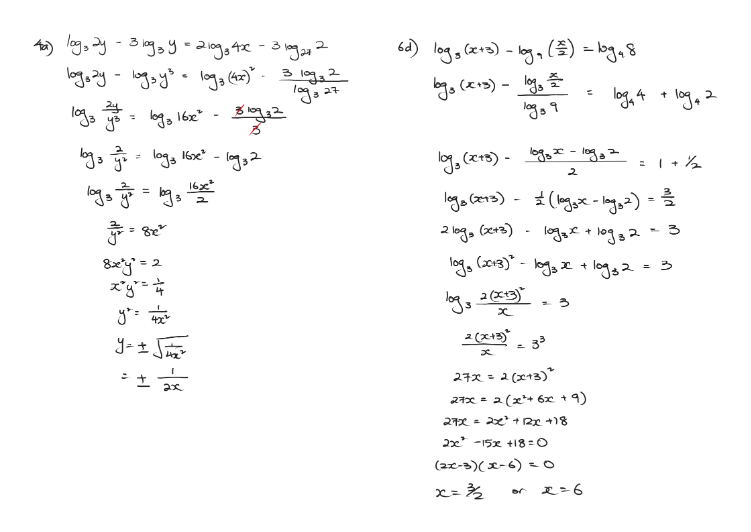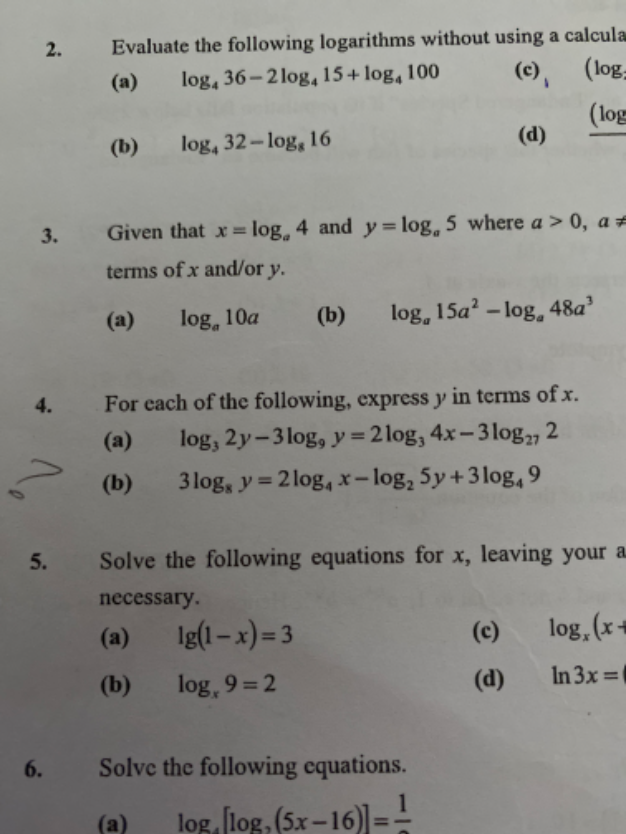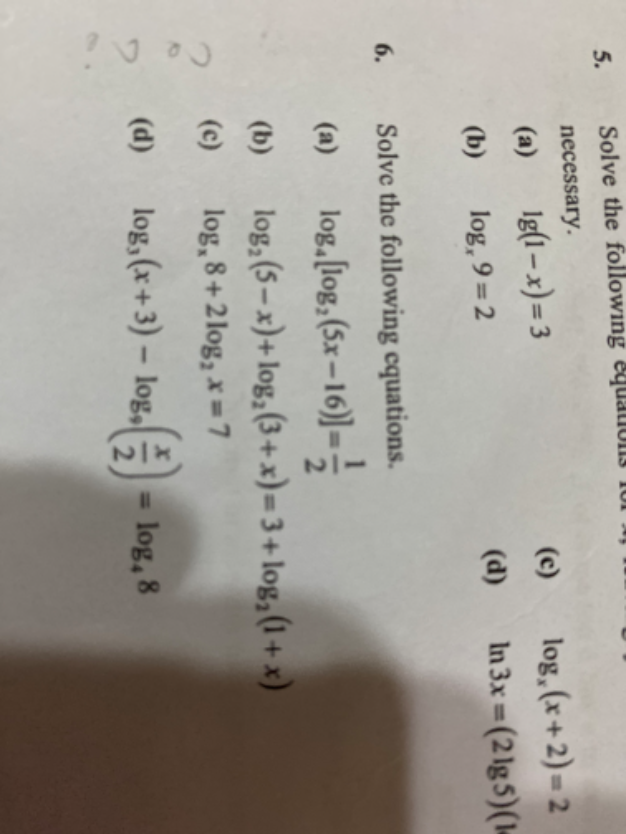# QuestionKindly solve q4a&b and q6 c&d. Thanks

Solution for 6(c)

logx 8+ 2 log2 x = 7

(log2 8) /  (log2 x )  +  2 log2 x = 7

3 / (log2 x)  +  2 log2 x = 7

Multiply both sides by  log2 x  to remove it from the denominator on LHS

3  + 2 (log2 x)2  = 7log2 x

Let A = log2 x

2A2 – 7A + 3 = 0

(2A – 1) (A – 3) = 0

=>  A =  1/2                                      or    A   = 3

log2 x = 1/2  =>  x = √ 2                    log2 x  = 3 =>  x = 8

*****************************************************************

Check by substituting both x into the original equation:

logx 8+ 2 log2 x = 7

When   x = √ 2  ,

LHS = log√ 2  8 +  2 log2 √ 2  = 6  +  log2 (2)(1/2)x2 = 6 + 1  =  7 = RHS

(√ 2)(6) = 8

log2 (2) = 1

When x = 8 ,

LHS  = log8 8+ 2 log2 8  = 1 + log2  (8)2 = 1 + 6 = 7 = RHS

(2)6 =  (23 ) = 64

that is the part when i multiply both side, i got 3 log2 X and that was wrong it has to be 4 logx X. thanks Sushi88

1 Reply 0 LikesYou need to upload better quality pictures.

2 Replies 1 LikeCan see better now?

1 Reply 0 Likes

how about 6c? thanks , tried 6 times, still cannot get it.

2 Replies 0 Likes6c question is logx 8 + 2 log2 X =7

1 Reply 0 Likes

Solution for 6(c)

logx 8+ 2 log2 x = 7

(log2 8) /  (log2 x )  +  2 log2 x = 7

3 / (log2 x)  +  2 log2 x = 7

Multiply both sides by  log2 x  to remove it from the denominator on LHS

3  + 2 (log2 x)2  = 7log2 x

Let A = log2 x

2A2 – 7A + 3 = 0

(2A – 1) (A – 3) = 0

=>  A =  1/2                                      or    A   = 3

log2 x = 1/2  =>  x = √ 2                    log2 x  = 3 =>  x = 8

*****************************************************************

Check by substituting both x into the original equation:

logx 8+ 2 log2 x = 7

When   x = √ 2  ,

LHS = log√ 2  8 +  2 log2 √ 2  = 6  +  log2 (2)(1/2)x2 = 6 + 1  =  7 = RHS

(√ 2)(6) = 8

log2 (2) = 1

When x = 8 ,

LHS  = log8 8+ 2 log2 8  = 1 + log2  (8)2 = 1 + 6 = 7 = RHS

(2)6 =  (23 ) = 64

that is the part when i multiply both side, i got 3 log2 X and that was wrong it has to be 4 logx X. thanks Sushi88

1 Reply 0 Likes

Answer for 4a is y=1/16x^4

4b y=(27x/5)^1/2

6d is correct x=1.5 or 6

Can you clarify 4a again?  Thanks for your help

0 Replies 1 Like

I have the same problem as Adwin, we cannot read your questions properly due to the blur image, hence we did not provide the solution.

Here’s my Solution for 4(b) blur-print question:

3 log8 y  = 2 log4 x – log2 5y + 3 log4 9

log2 y3 / log2 8 = (log2 x2  / log2 4 ) –  log2 5y  +  (log2 93  / log2 4 )

(1/3) log2 y =  (1/2) log2 x2 –  log2 5y  + (1/2) log2 93  <— [ 9 = (323 ) and logb(x y) = ylogb(x)]

log2 y  =  log2 x  –  log2 5y  +  log2 33

log2 y  = log2   [ (x)(27) / 5y ]

y2 = (27x) / 5

y =  ± √  [(27x)/5 ]

Since y cannot be negative,   –  √  [(27x)/5 ] is extraneous

Hence y =  √  [(27x)/5 ]

1 Reply 1 Like

Can help me on 5d? answer is 2.71 thanks

1 Reply 0 Likes

Yes, the blur picture misled both of us.  we saw the log9 as log3.

Solution for 4(a)

log3 2y – 3 log9 y  = 2log3 4x – 3log27 2

log3 2y – (log3 y3 ) / (log3  9) = log3 (4x)2  – 3 [ (log3 2) / (log3 27 ) ]

log3 2y –  (1/2) log3 y3  =  log3 16x2  –  log3 2

log [ 2y / (y)3/2]   =  log3 (16x2 /2)

2 / (y) 1/2  = 8x2

Square both sides to remove the square root of y,

4 / y = 64x4

y = 1 / 16x4

0 Replies 1 Like

4(a) is the same as Adwin’s solution

6(d) has a slight variation as we used different rules to get to the same end result.

Alternative solution for 6(d)

log3 (x + 3) – log9 (x/2) = log4 8

log3 (x + 3) – log3 (x/2) / log3 9 = log2 8  /  log2 4

log3 (x + 3) –  (1/2) log3 (x/2)  = 3/2

log3 [ (x + 3) / (x/2)1/2 ] = 3/2

(x + 3) / (x/2)1/2   = 33/2

Square both sides to get rid of the square root,

(x + 3)2  / (x/2) = 27

x2 + 6x + 9 = (27/2) x

2x2 + 12x + 18 – 27x = 0

2x2 – 15x + 18 = 0

(2x – 3)(x – 6 ) = 0

x = 3/2      or      x = 6

0 Replies 1 Like